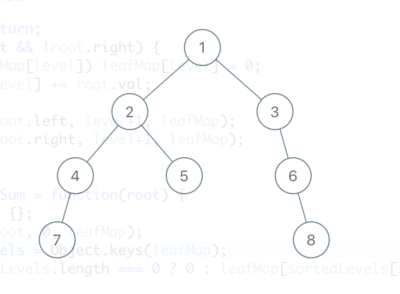Given a binary tree, return the sum of values of its deepest leaves.

Example 1:Input: root = [1,2,3,4,5,null,6,7,null,null,null,null,8]
Output: 15

Algorithm

• Traverse through the tree using a level variable (which tells you which level you’re at).
• When you reach a leaf, maintain a key map of level->sum.
• In the end, pick the highest key from the map and output the value (as result).

Also TIL, that Property order is predictable in JavaScript objects since ES2015. Quoting from this article “All properties that are integer indices appear first in the overall object property order and are sorted numerically.”. This means we don’t need to sort the leaf map (by key) before returning the result.

```/** * Definition for a binary tree node. * function TreeNode(val, left, right) { * this.val = (val===undefined ? 0 : val) * this.left = (left===undefined ? null : left) * this.right = (right===undefined ? null : right) * } */ /** * @param {TreeNode} root * @return {number} */   var calcLeafMap = function(root, level, leafMap) { if (!root) return; if (!root.left && !root.right) { if (!leafMap[level]) leafMap[level] = 0; leafMap[level] += root.val; } calcLeafMap(root.left, level+1, leafMap); calcLeafMap(root.right, level+1, leafMap); };   var deepestLeavesSum = function(root) { let leafMap = {}; calcLeafMap(root, 0, leafMap); let sortedLevels = Object.keys(leafMap); return leafMap[sortedLevels[sortedLevels.length-1]]; };```